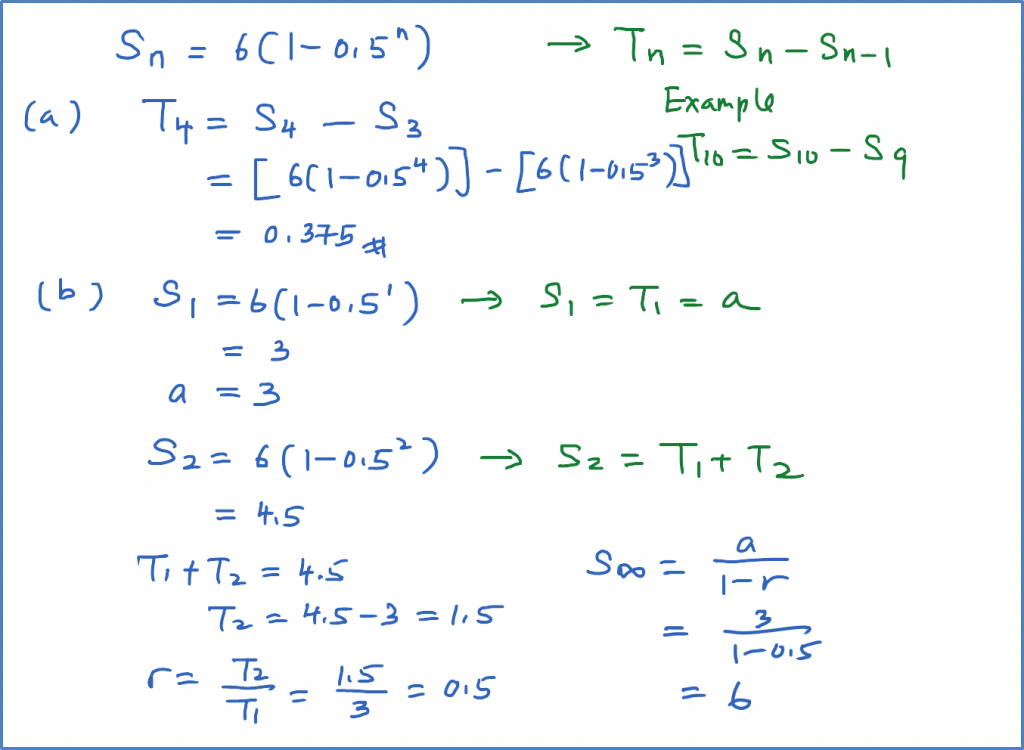# 1.5.3 Geometric Progressions, SPM Practice (Paper 1)

Question 11:
Express the recurring decimal 0.187187187 … as a fraction in its simplest form.

Solution:Question 12:

where p is a positive integer. If q = 0.1 and a + b + c are the first three terms of a geometric progression, state the value of a, b and c in decimal form. Hence find the value of p.

Solution:Question 13:
The sum of the first n term of a geometry progression is given by 6(1 – 0.5n ). Find
(a) The fourth term of the progression,
(b) The sum to infinity of the progression.

Solution:Question 14:
A gardener has the task of digging an area of 800 m2. On the first day he digs an area of 10 m2. On each successive day he digs an area of 1.2 times the area that he dug the previous day, until the day when the task is completed. Find the number of days needed to complete the task.

Solution: